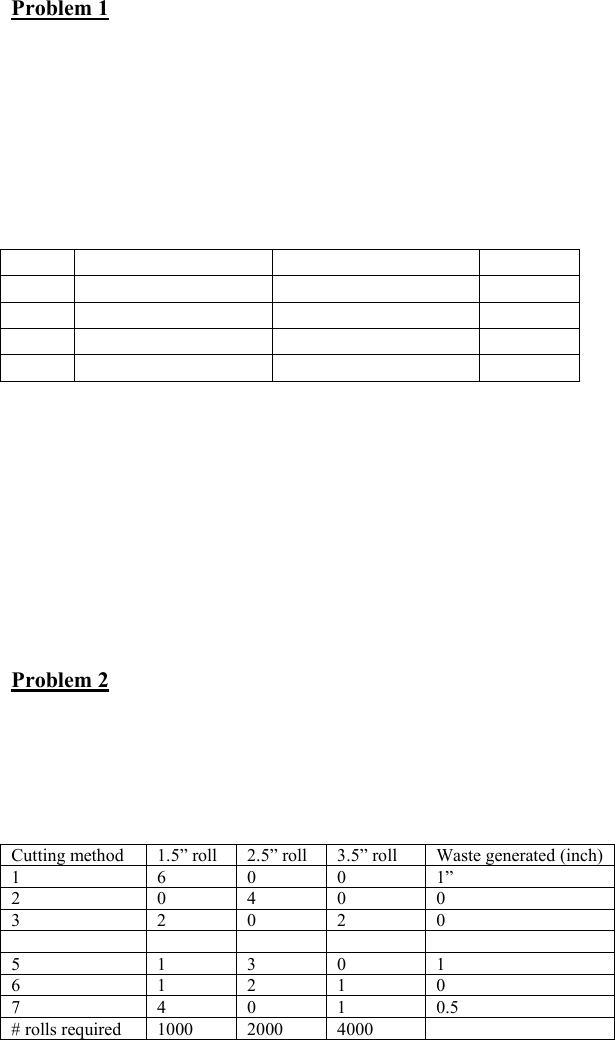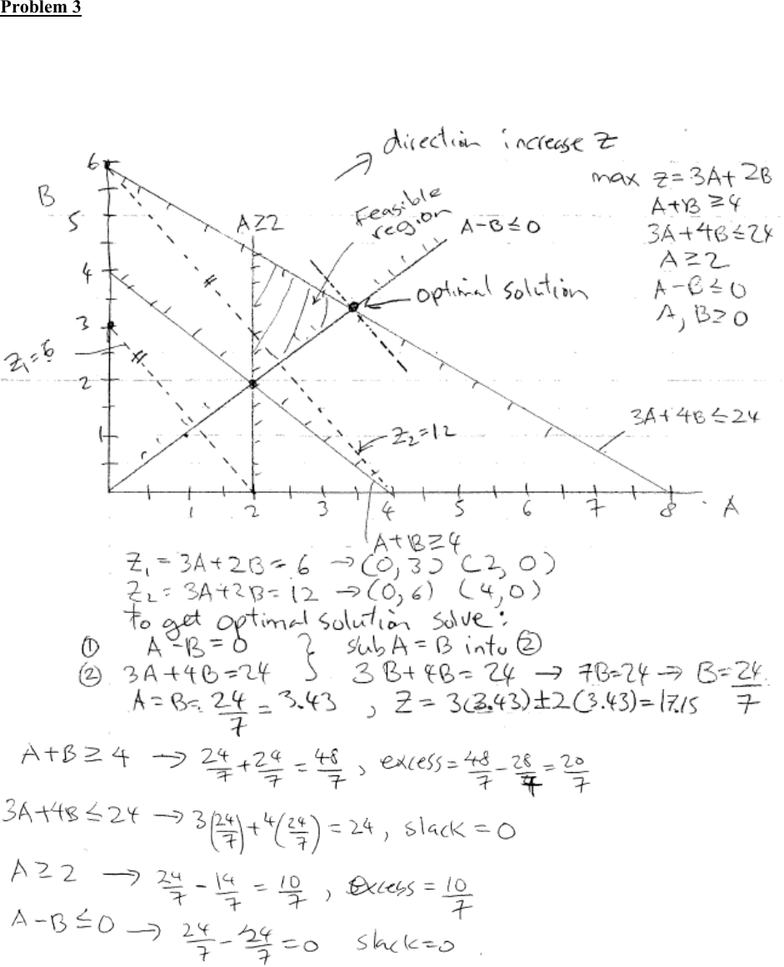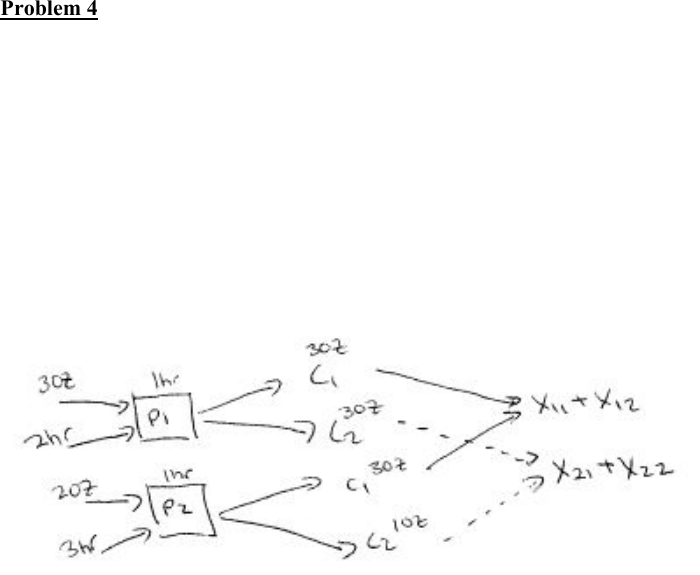Study Guides (390,000)
CA (150,000)
UTSC (10,000)
Midterm

MGOC10H3 Study Guide - Midterm Guide: Expected Return, Feasible Region, Graph PaperExam

Department
Management
Course Code
MGOC10H3
Professor
Vinh Quan
Study Guide
Midterm

This preview shows pages 1-3. to view the full 9 pages of the document.1
Department of Management, UTSC
MGOC10 Analysis for Decision Making
Practice Problem Set 1 Solution
Problem 1
Mutual Co is considering investing in four bonds. \$1M is available for investment, all of which is to be used. The
expected return, the worst-case annual return for each bond, and the “duration” of each bond is shown. The worst-
case return of the bond portfolio must be at least 8%. The average duration of the portfolio must be at most 6. For
example, a portfolio that invested \$600K in bond 1 and \$400K in bond 4 would have an average duration of
[\$600K(3) + 400K(9)/\$1M ] = 5.4. At most 40% of the total amount invested can be invested in bond 1. The total
amount invested in bond 2 cannot be more than 1/3 of the total amount invested in bonds 3 and 4 combined. The
amount invested in bond 3 cannot be \$50,000 more or less than the amount invested in bond 1. The % of money
invested in bond 4 must be equal to the % of money invested in bond 1 and 2 combined. Formulate an LP to help
Mutual to maximize the expected return on investments. (10 marks)
Bond
Expected return %
Worst case return %
Duration
1
13
6
3
2
8
8
4
3
12
10
7
4
14
9
9
Let xi = amount invested in bond i (assume entire \$1,000,000 invested since all investments are always profitable)
max z = .13x1 + .08x2 + .12x3 + .14x4
06x1 + .08x2 + .10x3 + .09x4.08(x1 + x2 + x3 + x4)
x1.4(x1 + x2 + x3 + x4) or x1 400,000
x1 + x2 + x3 + x4 = 1,000,000
3x2 (x3 + x4)
x3 x1 + 50,000 and x3 x1 50,000
x4/1,000,000 = (x1 + x2)/1,000,000
(3x1 + 4x2 + 7x3 + 9x4)/ (x1 + x2 + x3 + x4) 6
x1, x2, x3, x40
Problem 2
ABC Paper Co. produces rolls of paper for use in adding machines, desk calculators, and cash registers. The rolls
which are 200 feet long, are produced in widths of 1.5”, 2.5” and 3.5”. Raw materials are 200-foot 10-inch wide rolls
so ABC must cut the rolls into the final product sizes. There are 7 alternative cutting processes and the amount of each
product size generated from using each process is shown. ABC would like to limit the total amount of waste produced
from all processes to be at most 1500”. Formulate a LP model to help ABC minimize the number 10-inch rolls used to
make the 3 products. (5 marks)
Cutting method
1.5 roll
2.5 roll
Waste generated (inch)
1
6
0
1”
2
0
4
0
3
2
0
0
4
0
1
0.5
5
1
3
1
6
1
2
0
7
4
0
0.5
# rolls required
1000
2000
xi = # of 10-inch rolls of paper processed by cutting method i.
Min Z = x1 + x2 + x3 + x4 + x5 + x6 + x7
6x1 + 2x3 + x5 + x6 + 4x7 ≥ 1000 (1.5” rolls produced)
4x2 + x4 + 3x5 + 2x6 ≥ 2000 (2” rolls produced)
2x3 + 2x4 + x6 + x7 ≥ 4000 (3.5” rolls produced)
x1 + 0.5x4 + x5 + .5x7 ≤ 1500 (limit waste)
all variables ≥ 0

Only pages 1-3 are available for preview. Some parts have been intentionally blurred.2
Problem 3
MAX Z = 3A + 2B
s.t. A + B ≥ 4, 3A + 4B ≤ 24, A ≥ 2, A B ≤ 0, A, B ≥ 0
(a) Solve the problem the graphical method presented in class using the graph paper provided. Put A on X-axis.
Clearly mark the feasible region and direction of increase/decrease of Z and state what the optimal solution is.
Compute the slack or surplus for each constraint. (9 marks)
(b) Determine range of objective function coefficient for A for which the current solution will remain optimal. (7
marks)
Objective: Z = 3A + 2B get B = Z/2 c1/2A, slope = c1/2
Constraint 2: 3A + 4B ≤ 24 get B = 6 – 3/4A, slope = -3/4
Want slope objective steeper than slope Constraint : c1/2 ≤ -3/4 → – c1 -6/4 get c1 ≥ 1.5
As c1 → ∞ slope objective = - ∞ and solution in (a) still optimal. Therefore range is 1.5 ≤ c1 ≤ ∞

Only pages 1-3 are available for preview. Some parts have been intentionally blurred.3
(c) Determine the new optimal solution if 3A + 4B ≤ 24 became 3A + 4B ≤ 25. What is dual price for this constraint?
(6 marks)
if 3A + 4B ≤ 24 became 3A + 4B ≤ 25 the constraint shifts upwards to right and it affects optimal solution.
Solve 3A + 4B = 25 and A = B to get new optimal solution A* = B* = 25/7 = 3.571
New objective value = 3A + 2B = 3(25/7) + 2(25/7) = 125/7 = 17.86,
Old objective value = 3(24/7) + 2(24/7) = 120/7 = 17.15
Z increase by 5/7 = 0.71 for 1 unit increase in RHS therefore dual price = 0.71
Problem 4
ABC Drugs manufactures two drugs: 1 and 2. The drugs are produced by blending two chemicals: 1 and 2. By
weight, drug 1 must contain at least 65% chemical 1, and drug 2 must contain at least 55% of chemical 1. Drug 1 sells
for \$6/oz, and drug 2 sells for \$4/oz. Chemicals 1 and 2 can be produced via two production processes. Running
process 1 for an hour requires 3 oz of raw material and 2 hours skilled labor and yields 3 oz of each chemical.
Running process 2 for an hour requires 2 oz of raw material and 3 hours of skilled labor and yields 3 oz of chemical 1
and 1 oz of chemical 2. A total of 120 hours of skilled labor and 100 oz of raw material are available. Formulate an
LP that can be used to maximize ABC’s sales revenues. (10 marks)
p1, p2 = hours ran on process 1, 2
C1, C2 = ounces of chemical 1, 2 produced
Xij = ounces of chemical i used in drug j
Max Z = 6(x11 + x21) + 4(x12 + x22)
St. 3p1 + 2p2 ≤ 100 (raw material)
2p1 + 3p2 ≤ 120 (labor)
3p1 + 3p2 = C1 (chemical 1 produced)
3p1 + 1p2 = C2 (chemical 2 produced)
X11 + X12 ≤ C1 (chemical 1 used in drugs 1, 2)
X21 + X22 ≤ C2 (chemical 2 used in drugs 1, 2)
X11 ≥ .65 (X11 + X21) (proportion of chemicals in drug 1)
X12 ≥ .55 (X12 + X22) (proportion of chemicals in drug 2)
All VAR ≥ 0
You're Reading a Preview

Unlock to view full version